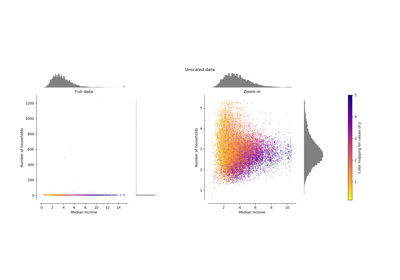# sklearn.preprocessing.MaxAbsScaler¶

class sklearn.preprocessing.MaxAbsScaler(copy=True)[source]

Scale each feature by its maximum absolute value.

This estimator scales and translates each feature individually such that the maximal absolute value of each feature in the training set will be 1.0. It does not shift/center the data, and thus does not destroy any sparsity.

This scaler can also be applied to sparse CSR or CSC matrices.

New in version 0.17.

Parameters: copy : boolean, optional, default is True Set to False to perform inplace scaling and avoid a copy (if the input is already a numpy array). scale_ : ndarray, shape (n_features,) Per feature relative scaling of the data. New in version 0.17: scale_ attribute. max_abs_ : ndarray, shape (n_features,) Per feature maximum absolute value. n_samples_seen_ : int The number of samples processed by the estimator. Will be reset on new calls to fit, but increments across partial_fit calls.

maxabs_scale
Equivalent function without the estimator API.

Notes

NaNs are treated as missing values: disregarded in fit, and maintained in transform.

For a comparison of the different scalers, transformers, and normalizers, see examples/preprocessing/plot_all_scaling.py.

Examples

>>> from sklearn.preprocessing import MaxAbsScaler
>>> X = [[ 1., -1.,  2.],
...      [ 2.,  0.,  0.],
...      [ 0.,  1., -1.]]
>>> transformer = MaxAbsScaler().fit(X)
>>> transformer
MaxAbsScaler(copy=True)
>>> transformer.transform(X)
array([[ 0.5, -1. ,  1. ],
[ 1. ,  0. ,  0. ],
[ 0. ,  1. , -0.5]])


Methods

 fit(X[, y]) Compute the maximum absolute value to be used for later scaling. fit_transform(X[, y]) Fit to data, then transform it. get_params([deep]) Get parameters for this estimator. inverse_transform(X) Scale back the data to the original representation partial_fit(X[, y]) Online computation of max absolute value of X for later scaling. set_params(**params) Set the parameters of this estimator. transform(X) Scale the data
__init__(copy=True)[source]
fit(X, y=None)[source]

Compute the maximum absolute value to be used for later scaling.

Parameters: X : {array-like, sparse matrix}, shape [n_samples, n_features] The data used to compute the per-feature minimum and maximum used for later scaling along the features axis.
fit_transform(X, y=None, **fit_params)[source]

Fit to data, then transform it.

Fits transformer to X and y with optional parameters fit_params and returns a transformed version of X.

Parameters: X : numpy array of shape [n_samples, n_features] Training set. y : numpy array of shape [n_samples] Target values. X_new : numpy array of shape [n_samples, n_features_new] Transformed array.
get_params(deep=True)[source]

Get parameters for this estimator.

Parameters: deep : boolean, optional If True, will return the parameters for this estimator and contained subobjects that are estimators. params : mapping of string to any Parameter names mapped to their values.
inverse_transform(X)[source]

Scale back the data to the original representation

Parameters: X : {array-like, sparse matrix} The data that should be transformed back.
partial_fit(X, y=None)[source]

Online computation of max absolute value of X for later scaling. All of X is processed as a single batch. This is intended for cases when fit is not feasible due to very large number of n_samples or because X is read from a continuous stream.

Parameters: X : {array-like, sparse matrix}, shape [n_samples, n_features] The data used to compute the mean and standard deviation used for later scaling along the features axis. y Ignored
set_params(**params)[source]

Set the parameters of this estimator.

The method works on simple estimators as well as on nested objects (such as pipelines). The latter have parameters of the form <component>__<parameter> so that it’s possible to update each component of a nested object.

Returns: self
transform(X)[source]

Scale the data

Parameters: X : {array-like, sparse matrix} The data that should be scaled.

## Examples using sklearn.preprocessing.MaxAbsScaler¶Compare the effect of different scalers on data with outliers